# Samacheer Kalvi 10th Maths Solutions Chapter 2 Numbers and Sequences Ex 2.2

## Tamilnadu Samacheer Kalvi 10th Maths Solutions Chapter 2 Numbers and Sequences Ex 2.2

10th Maths Exercise 2.2 Samacheer Kalvi Question 1.
For what values of natural number n, 4n can end with the digit 6?
Solution:
4n = (2 × 2)n = 2n × 2n
2 is a factor of 4n.
So, 4n is always even and end with 4 and 6.
When n is an even number say 2, 4, 6, 8 then 4n can end with the digit 6.
Example:
42 = 16
43 = 64
44 = 256
45 = 1,024
46 = 4,096
47 = 16,384
48 = 65, 536
49 = 262,144

Ex 2.2 Class 10 Samacheer Question 2.
If m, n are natural numbers, for what values of m, does 2n × 5m ends in 5?
2n is always even for any values of n.
[Example. 22 = 4, 23 = 8, 24 = 16 etc]

5m is always odd and it ends with 5.
[Example. 52 = 25, 53 = 125, 54 = 625 etc]
But 2n × 5m is always even and end in 0.
[Example. 23 × 53 = 8 × 125 = 1000
22 × 52 = 4 × 25 = 100]
? 2n × 5m cannot end with the digit 5 for any values of m.

Exercise 2.2 Class 10 Maths Samacheer Question 3.
Find the H.C.F. of 252525 and 363636.
Solution:
To find the H.C.F. of 252525 and 363636
Using Euclid’s Division algorithm
363636 = 252525 × 1 + 111111
The remainder 111111 ? 0.
? Again by division algorithm
252525 = 111111 × 2 + 30303
The remainder 30303 ? 0.
? Again by division algorithm.
111111 = 30303 × 3 + 20202
The remainder 20202 ? 0.
? Again by division algorithm
30303 = 20202 × 1 + 10101
The remainder 10101 ? 0.
? Again using division algorithm
20202 = 10101 × 2 + 0
The remainder is 0.
? 10101 is the H.C.F. of 363636 and 252525.

10th Maths Exercise 2.2 Question 4.
If 13824 = 2a × 3b then find a and b.
Solution:
If 13824 = 2a × 3b
Using the prime factorisation tree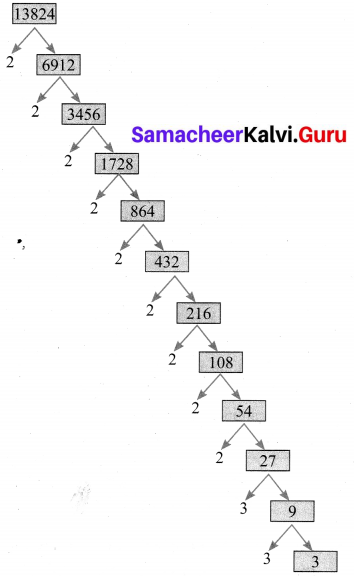13824 = 2 × 2 × 2 × 2 × 2 × 2 × 2 × 2 × 2 × 3 × 3 × 3
= 29 × 33 = 2a × 3b
? a = 9, b = 3.

10th Maths 2.2 Exercise Question 5.
If p1x1 × p2x2 × p3x3 × p4x4 = 113400 where p1, p2, p3, p4 are primes in ascending order and x1, x2, x3, x4 are integers, find the value of P1, P2, P3, P4 and x1, x2, x3, x4.
Solution:
If p1x1 × p2x2 × p3x3 × p4x4 = 113400
p1, p2, p3, P4 are primes in ascending order, x1, x2, x3, x4 are integers.
using Prime factorisation tree.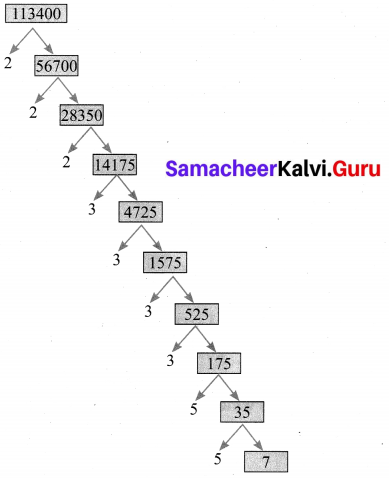113400 = 2 × 2 × 2 × 3 × 3 × 3 × 3 × 5 × 5 × 7
= 23 × 34 × 52 × 7
= p1x1 × p2x2 × p3x3 × p4x4
? p1= 2, p2 = 3, p3 = 5, p4 = 7, x1 = 3, x2 = 4, x3 = 2, x4 = 1.

10th Maths Exercise 2.2 In Tamil Question 6.
Find the L.C.M. and H.C.F. of 408 and 170 by applying the fundamental theorem of arithmetic.
Solution:
408 and 170.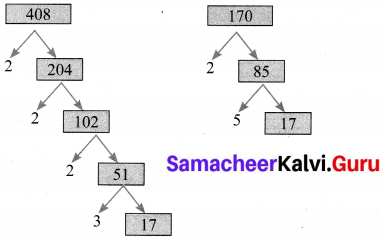408 = 23 × 31 × 171
170 = 21 × 51 × 171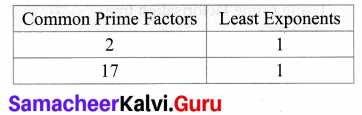? H.C.F. = 21 × 171 = 34.
To find L.C.M, we list all prime factors of 408 and 170, and their greatest exponents as follows.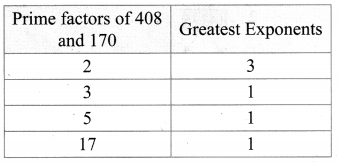? L.C.M. = 23 × 31 × 51 × 171
= 2040.

10th Maths Ex 2.2 Question 7.
Find the greatest number consisting of 6 digits which is exactly divisible by 24, 15, 36?
Solution:
To find L.C.M of 24, 15, 36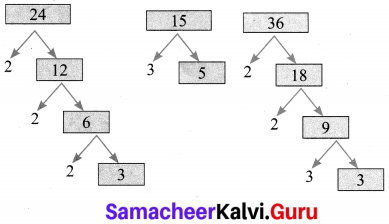24 = 23 × 3
15 = 3 × 5
36 = 22 × 32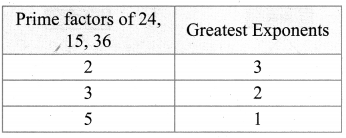? L.C.M = 23 × 32 × 51
= 8 × 9 × 5
= 360
If a number has to be exactly divisible by 24, 15, and 36, then it has to be divisible by 360. Greatest 6 digit number is 999999.
Common multiplies of 24, 15, 36 with 6 digits are 103680, 116640, 115520, …933120, 999720 with six digits.
? The greatest number consisting 6 digits which is exactly divisible by 24, 15, 36 is 999720.

10th Maths 2.2 Question 8.
What is the smallest number that when divided by three numbers such as 35, 56 and 91 leaves remainder 7 in each case?
Find the L.C.M of 35, 56, and 91
35 – 5 × 7 56
56 = 2 × 2 × 2 × 7
91 = 7 × 13
L.C.M = 23 × 5 × 7 × 13
= 3640
Since it leaves remainder 7
The required number = 3640 + 7
= 3647
The smallest number is = 3647

10th Maths Exercise 2.2 Answers Question 9.
Find the least number that is divisible by the first ten natural numbers.
Solution:
The least number that is divisible by the first ten natural numbers is 2520.
Hint:
1,2, 3,4, 5, 6, 7, 8,9,10
The least multiple of 2 & 4 is 8
The least multiple of 3 is 9
The least multiple of 7 is 7
The least multiple of 5 is 5
? 5 × 7 × 9 × 8 = 2520.
L.C.M is 8 × 9 × 7 × 5
= 40 × 63
= 2520